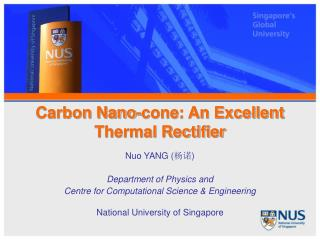# Carbon Nano-cone: An Excellent Thermal Rectifier - PowerPoint PPT PresentationDownload PresentationCarbon Nano-cone: An Excellent Thermal Rectifier

Carbon Nano-cone: An Excellent Thermal Rectifier
Download Presentation## Carbon Nano-cone: An Excellent Thermal Rectifier

- - - - - - - - - - - - - - - - - - - - - - - - - - - E N D - - - - - - - - - - - - - - - - - - - - - - - - - - -
##### Presentation Transcript

1. Carbon Nano-cone: An Excellent Thermal Rectifier Nuo YANG (杨诺) Department of Physics and Centre for Computational Science & Engineering National University of Singapore

2. Acknowledgement • Collaborators • Professor Dr. Baowen LI • National University of Singapore. • NUS Graduate School for Integrative Sciences and Engineering. • Dr. Gang ZHANG • Institute of Microelectronics, Singapore

3. Outline • Introduction • Thermal rectifier • Carbon Nano-cone • Model & Method • Results and Explanations • Conclusion

4. Introduction • Electrical rectifiers and transistors changed our life • Phononics (thermal) devices • phonon is used to carry and process information • broad applications for heat control/management • energy saving • Thermal Rectifier plays the most central role in phononics devices

5. Theory Models of Thermal Rectifier • Morse on-site potential • Controlling the Energy Flow in Nonlinear Lattices: A Model for a Thermal Rectifier, M. Terraneo, M. Peyrard, and G. Casati, Phys. Rev. Lett., 88, 094302 (2002). • Frenkel-Kontorova (FK) Model, • Thermal Diode: Rectification of Heat Flux, B. Li, L. Wang, and G. Casati, Phys. Rev. Lett. 93, 184301 (2004). • FK + Fermi-Pasta- Ulam (FPU) Model, • Interface Thermal Resistance between Dissimilar Anharmonic Lattices, B. Li, J. Lan, and L. Wang, Phys. Rev. Lett. 95, 104302 (2005). • FK Model • Asymmetric Heat Conduction in Nonlinear Lattices, B. Hu, L. Yang, and Y. Zhang, Phys. Rev. Lett., 97, 124302 (2006).

6. J+ • A Graded Mass Chain with FPU • Thermal rectification and negative differential thermal resistance in lattices with mass gradient, N. Yang, N. Li, L. Wang and B. Li, Phys. Rev. B 76, 020301(R) (2007) • Conclusion for Theory Models of Thermal Rectifier • High the rectifications: R~ 100,000% • Difficult to achieve experimentally • Length dependence J-

7. Carbon Nanotube Thermal Rectifier • The first solid-state thermal rectifier, carbon or boron nitride nanotubes with non-uniform axial mass distribution, was reported experimentally by C. W. Chang, D. Okawa, A. Majumdar, and A. Zettl. [a] • G. Wu and B. Li also studied the rectification in carbon nanotube junctions by using molecular dynamics method. [b] • However, the rectifications (~ 7% for [a] and ~20% for [b]) limit the application of the nanotube thermal rectifier. • Results in [b] showed the rectification was dependent on the length of nanotube [a] C. W. Chang, D. Okawa, A. Majumdar, and A. Zettl, SCIENCE, 314, 1121 (2006). [b] G. Wu and B.Li, Phys. Rev. B 76, 085424 (2007).

8. Carbon nano-cone • Observed by Maohui Ge and Klaus Sattler,1994.[a] • Characterized by cone angle, five possible angles are 19, 39, 60, 85 and 113 degrees [b] • High Asymmetry in geometry • Semiconductor [c] [a] M. Ge and K. Sattler, Chem. Phys. Lett., 220, 192 (1994) [b] T. W. EBBESEN, Acc. Chem. Res., 31, 558-566 (1998) [c] J.-C.Charlier and G.-M. Rignanese Phys. Rev. Lett., 86, 5970 (2001).

9. Model & Method • Classical molecular dynamics simulations used • No. of Atoms : 2,577 ~20,155 • A Morse bond and a harmonic cosine angle for C-C bonding interaction, which include both two-body and three-body potential terms [a] • Nosé-Hoover heat bathes are used • The heat flux is defined as the energy transported along the surface in unit time [b]: • where is εi local site energy, Fij is two-body force, and Fj(ijk) is three-body force [a] R. E. Tuzun, D. W. Noid , B. G. Sumpter, and R. C. Merkle, Nanotech. 7 (3), 241-248. (1996) [b] P. K. Schelling; S. R. Phillpot; and P. Keblinski, Phys. ReV. B, 65, 144306 (2002).

10. Results and Explanations Figure. (a) Rectifications for the homogenous mass carbon nanocone. (b) Heat flux J versus Δ. (c) Temperature Profile of nanocone for | Δ |=0.5. Here, N=40, and T0=300 K.

11. The factors which affect the rectifications • Temperature effect Figure (a) and (b) Rectifications and heat fluxes of carbon nanocone at different temperature, T0, with fixed N=40 layers and | Δ |=0.5.

12. Size independence Figure. (c) and (d) Rectifications and heat fluxes for different length of carbon nanocone, with fixed T0= 300K, and . Here N changes from 20 layers to 100 layers . The rectification is about 100%.

13. Mechanics Figure. Phonon Spectra of atoms in layers close to the top and bottom. (a) and (b)T0=50K Upper panel, the bottom heat bath is at high temperature (Δ=0.5) which corresponds to big heat flux. Lower panel, Δ =-0.5 which corresponds to small heat flux. * J. Shiomi and S. Maruyama, Non-Fourier heat conduction in a single-walled carbon nanotube: Classical molecular dynamics simulations, Phys. Rev. B, 73, 205420 (7 pages), (2006).

14. Figure. Phonon Spectra of atoms in layers close to the top and bottom. (a) and (b) T0=50K (c) and (d)T0=300K; Upper panel, the bottom heat bath is at high temperature (Δ=0.5) which corresponds to big heat flux. Lower panel, Δ =-0.5 which corresponds to small heat flux.

15. Conclusion • An excellent thermal rectifier realized with carbon nanocone is proposed. • Temperature effect and length independence are shown. • Physical mechanism for the carbon nanocone thermal diode is understood by the match/mismatch phonon’sDOS spectra. Thanks